# Area, perimeter and volume lesson plan

 QCA Unit Mathematics at KS 4, Unit : Area, Perimeter and Volume. Year Group Year 8 Number in class 30 Time for lesson 1 hour No. of computers 15 (minimum).

Aims/Learning Outcomes: Learners will be able to estimate/calculate the Area and Perimeter of 2D shapes and Volume of 3D shapes.

All:. estimate/calculate the Area and Perimeter of 2D shapes and Volume of 3D shapes.

Some: estimate/calculate the Area and Perimeter of more complex 2D shapes.

Keywords: area, cuboid, cylinder, perimeter, 2D, 3D, multiple, divide, whole and mixed numbers, prism,quadrilaterals, surface, triangle, volume.

Resources:interactive whiteboard, pre-prepared presentation and questions on calculating area, perimeter, and volume.

Differentiation: By learning outcome, and differentiated tasks activities. Group work at different levels where the tasks are simplified/extended in terms of content and/or language for specific groups, i.e. simpler multiplication problems for mental warm-up. Those in this class who are in lower ability Maths groups to be aided by teaching support staff as available.

Mental Maths Start Up.

Go through some mental warm up exercises involving the multiplication of whole and mixed numbers.

Introduction. Explain that the class are going to learn how to estimate/calculate the area and perimeter of 2D shapes and volume of some 3D shapes.

On the interactive whiteboard, explain the basic rules for these operations. Explain that there are various ways of writing down these conventions, and it is best to be aware of these as you may encounter them in different contexts

Area

For a square, the area is the measurement of one side squared. ( x by itself).

For a rectangle, the area is the height multiplied by the width. (h x w). or A=hw.

For a parallelogram, the area is the base multiplied by the height.

For a trapezoid, the area is 1 (B1 + B2) x height

2

For a triangle, the area is the half of the base multiplied by the height, A= bh

2

For a circle, the area is pr squared, or the radius x 3.14 squared.

Perimeter

For a square, the perimeter is the measurement of one side multiplied by 4.

For a rectangle, the perimeter is the height multiplied by 2 added to the width multiplied by 2.

For a parallelogram, the perimeter is the height multiplied by 2 added to the width multiplied by 2.

For a trapezoid, the perimeter is the height multiplied by 2 added to the width multiplied by 2.

For a triangle, the perimeter is side a added to side b added to side c.

Volume

For a cube, the volume is the height multiplied by the width multiplied by the length.

For a cuboid or rectangular solid, the volume s the height multiplied by the width multiplied by the length.

For a prism, the volume is the base x height.

For a cylinder, the volume is pr squared x height.

Main Activity. (Approximately 20 minutes). Give the class some practice in using these various formulae in calculating the area, perimeter and volume of shapes.

Area

1. Find the area of a square with sides of 7 cms.

2. Find the area of a square with sides of 19.5 cms.

3. Find the area of a rectangle with a height of 12 cms and a width of 11 cms.

4. Find the area of a rectangle with a height of 18 cms and a width of 14 cms.

5. Find the area of a triangle with a base of 14 cms and a height of 19 cms.

5. Find the area of a triangle with a base of 9 cms and a height of 12 cms.

6. Find the area of a triangle with a base of 14 cms and a height of 19 cms.

7. Find the area of a triangle with a base of 11.6 cms and a height of 15 cms.

8. Find the area of a football pitch with a length of 90 metres and width of 45 metres.

9. The regulation size of a full sized football association goal is 2.44 metres high and 7.32 metres wide. How big is the space that the goalkeeper must protect?

10. FA rules state that a full-sized pitch must a minimum of 90 metres and a maximum of 120 yards in length, and a minimum of 45 metres and maximum of 90 metres long. If a team play on a minimum size pitch one week, and a maximum size pitch the following week, what is the difference in the playing surfaces they have to cover?

8. a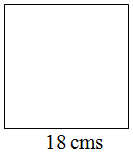Find the area of this square.

b.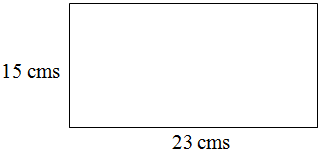Find the area of this shape.

c.Find the area of this shape.

d.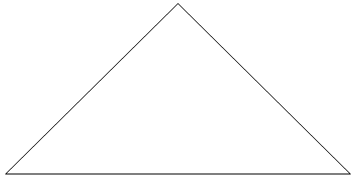This shape has a base of 18 cms and a height of 8 cms. What is its area?

e.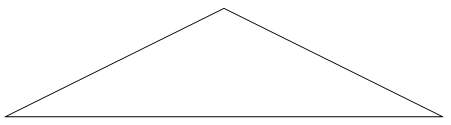This shape has a height of 11 cms and a base of 31 cms. What is its area?

f.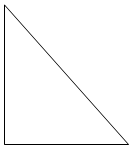This shape has a height of 17 cms and a base of 12 cms. What is its area?

Perimeter.

1. Find the perimeter of a square with sides of 9 cms.

2. Find the perimeter of a square with sides of 17.5 cms.

3. Find the perimeter of a rectangle with a height of 11 cms and a width of 14 cms.

4. Find the perimeter of a rectangle with a height of 19 cms and a width of 13 cms.

5. Find the perimeter of a triangle whose sides measure 11cms, 9cms, and 23cms.

6. Find the perimeter of a triangle whose sides measure 18cms, 15cms, and 21cms.

6. Find the perimeter of a triangle whose sides measure 18cms, 15cms, and 21cms.

6. Find the perimeter of an equilateral triangle whose base measures 18cms.

7. Find the perimeter of an equilateral triangle whose base measures 23cms.

8. Find the perimeter of an equilateral triangle whose left side measures 14cms.

9. Find the perimeter of an equilateral triangle whose right side measures 18 cms.

10. Find the perimeter of an isosceles triangle whose right side measures 12 cms and whose base measures 5 cms.

11. Find the perimeter of an isosceles triangle whose right side measures 17.5 cms and whose base measures 26 cms.

12. Find the perimeter of an isosceles triangle whose right side measures 34 cms and whose base measures half of that.

13. Find the perimeter of a scalene triangle whose right side measures 13 cms, left side measures 11 cms, and whose base measures 9 cms.

14. Find the perimeter of a scalene triangle whose right side measures 9 cms, left side measures 12 cms, and whose base measures 5 cms.

15. Find the perimeter of a scalene triangle whose right side measures 7 cms, left side measures 13 cms, and whose base measures half of their total.

16. Find the perimeter of a football pitch with a length of 90 metres and a width of 45 metres.

Volume

1. Find the volume of a cube with sides of 8 cms.

2. Find the volume of a cube with sides of 81 cms.

3. Find the volume of a cube with sides of 1.5 cms.

4. Find the volume of a cuboid with a height of 11 cms, a width of 14 cms, and a length of 12 cms.

5. Find the volume of a cuboid with a height of 19 cms, a width of 13 cms, and a length of 15 cms.

6. Find the volume of a prism with an area of 19 cms, a height of 19 cms, and a length of 25 cms.

7. Find the volume of a prism with an area of 21 cms, a height of 18 cms and a length of 32 cms.

8. A Toblerone bar is a prism based on an equilateral triangle whose base measures 7 cms. If the bar is 30 cms, what volume of chocolate is in the Toblerone?

9. Toblerone market a new giant 40 cm long bar based on an isosceles triangle, whose right side measures 12 cms, and whose base measures 5 cms. What volume of chocolate needs to go into the new product?

10. A rival company are marketing a new chocolate in the shape of cylinder, with a area of 30 cms and a length of 35 cms. What volume of chocolate are they going to need to put in it?

Plenary. Check the answers and methods used by the class. Check that the questions embedded in text were correctly deciphered, i.e. those on the areas of football pitches and volumes of chocolate.

Assessment.

Relevant NC Level Descriptors.

For assessment purposes, successful completion of this lesson will enable pupils to achieve the following aspects of the National Curriculum Level Descriptors in Mathematics.

Level 4. They find perimeters of simple shapes and find areas…. This requirement should be met by the completion of the independent tasks in this lesson.

Level 5. They make sensible estimates of a range of measures in relation to everyday situations. Pupils understand and use the formula for the area of a rectangle. This requirement should be met by the completion of the independent tasks in this lesson, i.e. calculating the areas of rectangles and solving related problems in real life contexts.

Level 6. Pupils recognise and use common 2-D representations of 3-D objects. They know and use the properties of quadrilaterals… areas of plane rectilinear figures and volumes of cuboids when solving problems. This requirement should be met by the completion of the independent tasks in this lesson, i.e. calculating the volumes of cuboids etc.

Level 7. They calculate lengths, areas and volumes in plane shapes and right prisms. Pupils appreciate the imprecision of measurement and recognise that a measurement given to the nearest whole number may be inaccurate by up to one half in either direction. This requirement should be met by the completion of the independent tasks in this lesson, i.e. calculating the areas, volumes and perimeters of various plane and three-dimensional objects, to the nearest whole number or lowest terms.

Originally published on Essay.uk.com